Monday, September 27, 2021
Home > download jstse papers > JSTSE PREVIOUS YEAR QUESTION PAPER 2012-13

# JSTSE PREVIOUS YEAR QUESTION PAPER 2012-13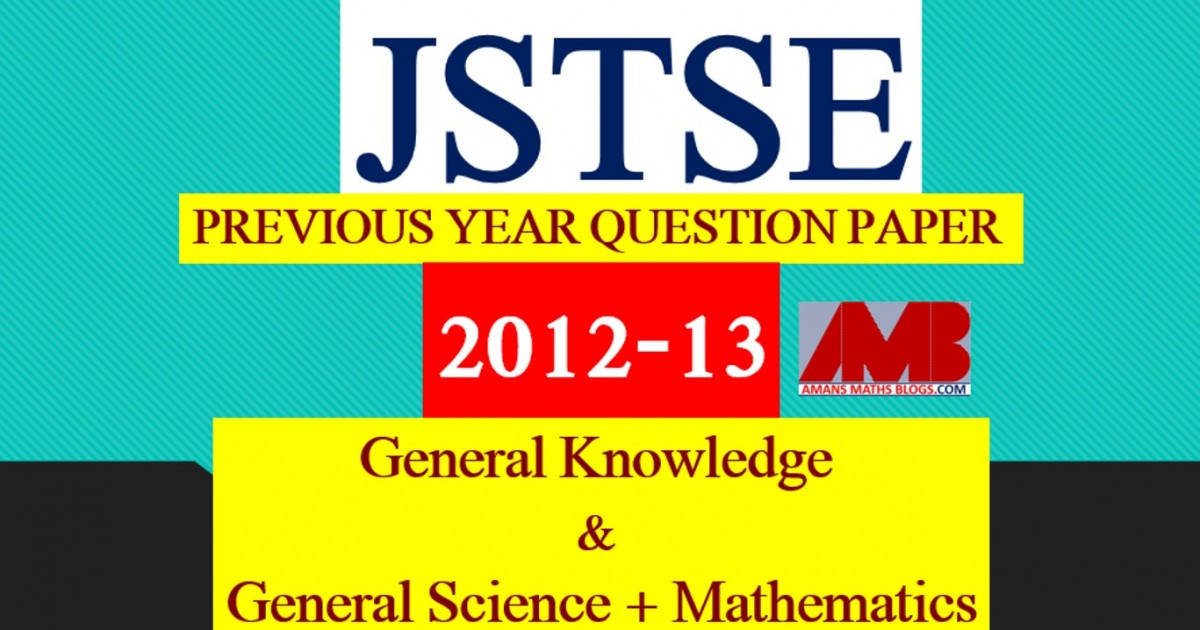# JSTSE PREVIOUS YEAR QUESTION PAPER 2012-13

Junior Science Talent Search Examination (JSTSE) is conducted by Science Branch of DTE of Education, Delhi every year usually in the month of January for the students of recognized schools of Delhi. In this post, you will get JSTSE Previous Year Question Paper 2012-13.

JSTSE
Previous Year Question Papers
2007-082008-092009-102010-112011-12
2012-132013-142014-152015-162016-17
2018-19PhysicsChemistryMathsBiology

The eligibility of JSTSE exam is any students of recognizing school studying in Class 9th in Govt/Public/KV/Navodaya etc and must have secured 65% marks in Class 8th.

Examination fee: – No examination fee is charged from students for appearing in this exam.
Type of Examination: – The examination consists of two papers.
I. General Knowledge
No. of Questions: 50       Marks : 50     Time Alloted: 50 min
50 50 50 min.
II . General Science and Mathematics
No. of Questions : 150      Marks : 150     Time Alloted: 150 min

No. of Scholarships:- There are 150 scholarships for JSTSE per year.

Read More : 129 Maths Short Tricks

## General Science + Mathematics

JSTSE Previous Year Question Papers 2012-13 Ques No 1:

If 6/(3√2 – 2√3) = 3√2 – a√6, then the value of a is

Options:

A. √2

B. –√2

C. 2√3

D. -2√3

JSTSE Previous Year Question Paper 2012-13 Ques No 2:

The value of (x + y)-1(x-1 + y-1) is

Options:

A. 1

B. (x + y)-2

C. x-1y-1

D. (xy)-1(x + y)-1

JSTSE Previous Year Question Papers 2012-13 Ques No 3:

Which of the following is equal to x?

Options:

A. x11/7 – x4/7

B. (x11/7)(x4/7)

C. 12√(x4)1/3

D. (√x4)1/2

JSTSE Previous Year Question Paper 2012-13 Ques No 4:

In the figure, AB and CD intersect at O. The value of x is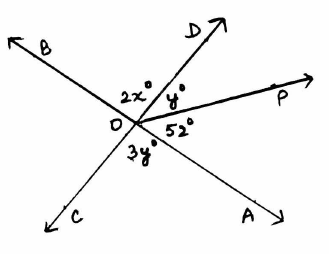Options:

A. 32

B. 42

C. 48

D. 96

JSTSE Previous Year Question Papers 2012-13 Ques No 5:

In the figure, AB = BD and AD = DC, then ∠BAC : ∠ACB isOptions:

A. 1 : 2

B. 2 : 1

C. 1 : 3

D. 3 : 1

JSTSE Previous Year Question Paper 2012-13 Ques No 6:

The perimeter of an equilateral triangle is 60 m. Then its area will be

Options:

A. 5√3 m2

B. 25√3 m2

C. 100√3 m2

D. 200√3 m2

JSTSE Previous Year Question Papers 2012-13 Ques No 7:

Area of rhombus whose one side is 13 cm and one of the diagonals is 10 cm is

Options:

A. 36 m2

B. 52 m2

C. 60 m2

D. 120 m2

JSTSE Previous Year Question Paper 2012-13 Ques No 8:

ABCDE is a pentagon inscribed in a circle with center O. Then the value of ∠ABC + ∠CDE will be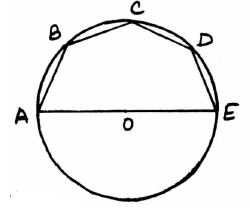Options:

A. 108 degree

B. 180 degree

C. 270 degree

D. 360 degree

JSTSE Previous Year Question Papers 2012-13 Ques No 9:

The area of the shaded region is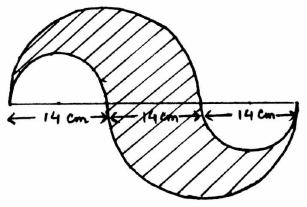Options:

A. 154 cm2

B. 231 cm2

C. 462 cm2

D. 616 cm2

JSTSE Previous Year Question Paper 2012-13 Ques No 10:

Square piece of side 2 cm are cut off from each corner of a square sheet of side 9 cm. The flaps of the sheet so formed are folded to form an open box. The volume of the box is

Options:

A. 20 cm3

B. 28 cm3

C. 50 cm3

D. 98 cm3

JSTSE Previous Year Question Papers 2012-13 Ques No 11:

If (a + 1/a)2 = 3, then the value of a3 + 1/a3 is

Options:

A. 0

B. -2√3

C. 3√3

D. 6√3

JSTSE Previous Year Question Paper 2012-13 Ques No 12:

If the polynomial (ax3 + 4x2 + 3x – 4) and (x3 – 4x + a) leave the same remainder when divided by (x – 3) then the value of ‘a’ is

Options:

A. 1

B. -1

C. 19/14

D. -5/14

JSTSE Previous Year Question Papers 2012-13 Ques No 13:

In a square PQRS, if P(1, 0), Q(4, 0) and S(1, 3) then the coordinates of point R will be

Options:

A. (4, 1)

B. (4, 4)

C. (3, 4)

D. (4, 3)

JSTSE Previous Year Question Paper 2012-13 Ques No 14:

In the figure, if O is the center of the circle, then ∠TRQ isOptions:

A. 25 degree

B. 35 degree

C. 45 degree

D. 70 degree

JSTSE Previous Year Question Papers 2012-13 Ques No 15:

The edges of a triangular board are 50 cm, 120 cm, 130 cm. The cost of painting it at the rate of Rs 900 per m2 is

Options:

A. Rs. 27

B. Rs. 270

C. Rs. 540

D. Rs. 27000

JSTSE Previous Year Question Paper 2012-13 Ques No 16:

Mean of all factors of 18 is

Options:

A. 4.25

B. 5.0

C. 6.5

D. 7.6

JSTSE Previous Year Question Papers 2012-13 Ques No 17:

In the figure, if O is the center of the circle, then the value of x is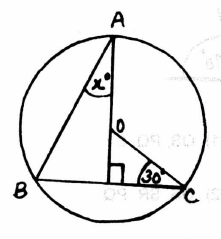Options:

A. 15

B. 30

C. 45

D. 60

JSTSE Previous Year Question Paper 2012-13 Ques No 18:

The distance of a point from the x-axis and the y-axis are 5 and 4 respectively. The coordinates of the point can be

Options:

A. (5, 4)

B. (5, 0)

C. (0, 4)

D. (4, 5)

JSTSE Previous Year Question Papers 2012-13 Ques No 19:

The volume of the two spheres is in the ratio of 125 : 64. Then the ratio of their surface areas will be

Options:

A. 5 : 4

B. 25 : 16

C. 16 : 25

D. 125 : 64

JSTSE Previous Year Question Paper 2012-13 Ques No 20:

In the figure, PS bisects angle P, PQ, QS, SR are in the ascending order areOptions:

A. QS, PQ, SR

B. QS, SR, PQ

C. PQ, QS, SR

D. SR, PQ, QS

JSTSE Previous Year Question Papers 2012-13 Ques No 21:

The mean marks of boys and girls in an examination are 60 and 65 respectively. If the mean marks of all the students in that examination is 62, then the ratio of the number of boys to that the number of girls is

Options:

A. 2 : 3

B. 3 : 2

C. 122 : 127

D. 5 : 62

JSTSE Previous Year Question Paper 2012-13 Ques No 22:

In the figure, AB || PQ and CD || PS, then ∠QPR is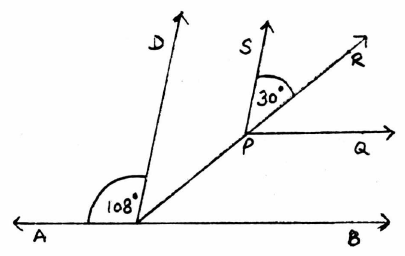Options:

A. 30 degree

B. 42 degree

C. 52 degree

D. 108 degree

JSTSE Previous Year Question Papers 2012-13 Ques No 23:

Autorikshaw fare in a city is Rs. 20 for first two kilometer and Rs. 6 per kilometer for subsequent distances covered. Taking the distance covered as x km and total fare as Rs. y, the linear equation in which expresses the above statement is

Options:

A. y = 6x + 8

B. y = 6x – 8

C. y = 6x + 20

D. y = 6x + 28

JSTSE Previous Year Question Paper 2012-13 Ques No 24:

If an angle is 24 degree more than half of its complement then the angle is

Options:

A. 14 degree

B. 38 degree

C. 46 degree

D. 76 degree

JSTSE Previous Year Question Papers 2012-13 Ques No 25:

20 circular plates each of radius 14 cm and thickness is 2 cm are placed one above the other to form a cylinderical solid. The total surface area will be

Options:

A. 2992 cm2

B. 3520 cm2

C. 4752 cm2

D. 24640 cm2

JSTSE Previous Year Question Paper 2012-13 Ques No 26:

ABCD is a parallelogram with AB = (2x + 6) cm, BC = 8 cm and CD = (x + 8) cm. The perimeter of the parallelogram ABCD is

Options:

A. 12 cm

B. 20 cm

C. 28 cm

D. 36 cm

JSTSE Previous Year Question Papers 2012-13 Ques No 27:

For the data (2, 9, x + 6, 2x + 3, 5, 10, 5) if mean is 7, then mode is

Options:

A. 3

B. 5

C. 9

D. 10

JSTSE Previous Year Question Papers 2012-13 Ques No 28:

ABCD is a rhombus in which altitude from D to side AB bisects AB. Then angle D of the rhombus is

Options:

A. 60 degree

B. 90 degree

C. 120 degree

D. 135 degree

JSTSE Previous Year Question Paper 2012-13 Ques No 29:

From the data (1, 4, 7, 16, 27, 29) if 29 is removed, probability of getting a prime number is

Options:

A. 1/2

B. 1/5

C. 2/5

D. 1/3

JSTSE Previous Year Question Papers 2012-13 Ques No 30:

PQRS is a square. T and U are respectively the mid-points of PS and QR. If O is the point of intersections of TU and QS and PQ = 8 cm, then area of ∆OTS is

Options:

A. 4 cm2

B. 8 cm2

C. 12 cm2

D. 16 cm2

JSTSE
Previous Year Question Papers
2007-082008-092009-102010-112011-12
2012-132013-142014-152015-162016-17
2018-19PhysicsChemistryMathsBiology
Get Online Maths Tuition by AMBiPi for Class 8 to 12